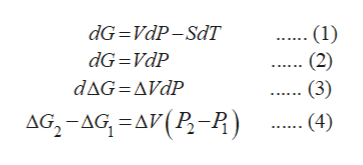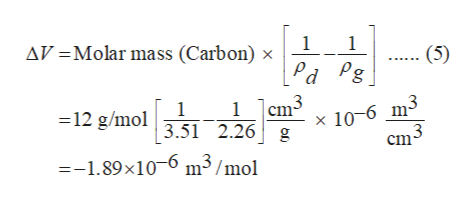Tired of studying thermodynamics, you decide that you will abandon chemical engineering and get rich quick by manufacturing diamond from graphite.It is known that the difference between the Gibbs free energy for diamond and graphite at normalroom temperatures (25° C) is 2866 J/mol, i.e.,:g (25°C, 1 atm) = gdiamond – ggraphite = 2866 J/molAlso, the density data of diamond and graphite at 25°C are also known as such:diamond = 3.51 g/cm3graphite = 2.26 g/cm3Using this information, estimate the pressure you would have to exert in order to have diamondand graphite be in equilibrium at room temperature.

Question

Tired of studying thermodynamics, you decide that you will abandon chemical engineering and get rich quick by manufacturing diamond from graphite.
It is known that the difference between the Gibbs free energy for diamond and graphite at normal
room temperatures (25° C) is 2866 J/mol, i.e.,:
g (25°C, 1 atm) = gdiamond – ggraphite = 2866 J/mol
Also, the density data of diamond and graphite at 25°C are also known as such:
diamond = 3.51 g/cm3
graphite = 2.26 g/cm3
Using this information, estimate the pressure you would have to exert in order to have diamond
and graphite be in equilibrium at room temperature.

Step 1

The fundamental equation for Gibb’s free energy change is given by equation (1). Since, the temperature remains constant in the process of conversion of Carbon (graphite) (initial state) to Carbon (diamond) (final state), therefore, equation (1) can be reduced to equation (2).

Let deltaG1 be the molar Gibb’s free energy change taking place when Carbon (graphite) converts to Carbon (diamond), which is 2866 J/mol at normal room temperatures (25° C). Let deltaG2 be the molar Gibb’s free energy change taking place when both these states are in equilibrium so that deltaG2 = 0.

Equation (3) can be deduced from equation (2) which on further simplification can be reduced to equation (4). Here, P2 is the pressure that is exerted to bring graphite and diamond in equilibrium at room temperature, P1 is initial pressure, i.e., 1 atm or 101325 Pa and deltaV is change in molar volume.help_outlineImage TranscriptionclosedG VdP-SdT (1) (2) (3) dG VdP dAG AVdP AG-AG A(P-P) (4) fullscreen
Step 2

Change in volume is calculated using equation (5) where ρd is density of diamond (3.51 g/cm3), ρg is density of graphite (2.26 g/cm3) and molar mass of carbon is 12 g/mol. Substitute the known values in...help_outlineImage Transcriptionclose1 AV Molar mass (Carbon) x 1 (5) Pd Pg 3 cm 1 =12 g/mol3.51 2.26 g 1 x 10-6 m- cm --1.89x10 m3/mol fullscreen

Want to see the full answer?

See Solution

Want to see this answer and more?

Our solutions are written by experts, many with advanced degrees, and available 24/7

See Solution
Tagged in

Chemical Engineering